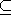# 3D Convex HullsReference Manual

Susan Hert and Stefan Schirra

A subset S3 is convex if for any two points p and q in the set the line segment with endpoints p and q is contained in S. The convex hull of a set S is the smallest convex set containing S. The convex hull of a set of points P is a convex polytope with vertices in P. A point in P is an extreme point (with respect to P) if it is a vertex of the convex hull of P.

CGAL provides functions for computing convex hulls in two, three and arbitrary dimensions as well as functions for testing if a given set of points in is strongly convex or not. This chapter describes the functions available for three dimensions.

### Assertions

The assertion flags for the convex hull and extreme point algorithms use CH in their names (e.g., CGAL_CH_NO_POSTCONDITIONS). For the convex hull algorithms, the postcondition check tests only convexity (if not disabled), but not containment of the input points in the polygon or polyhedron defined by the output points. The latter is considered an expensive checking and can be enabled by defining CGAL_CH_CHECK_EXPENSIVE .